﻿ 利用自适应混沌遗传粒子群算法反演瑞雷面波频散曲线
 石油地球物理勘探2019, Vol. 54Issue (6): 1217-1227  DOI: 10.13810/j.cnki.issn.1000-7210.2019.06.0050文章快速检索 高级检索

### 引用本文YANG Bo, XIONG Zhang-qiang, ZHANG Dazhou, YANG Zhen-tao. Rayleigh surface-wave dispersion curve inversion based on adaptive chaos genetic particle swarm optimization algorithm. Oil Geophysical Prospecting, 2019, 54(6): 1217-1227. DOI: 10.13810/j.cnki.issn.1000-7210.2019.06.005.### 作者简介张大洲, 湖南省长沙市岳麓区麓山南路932号中南大学地球科学与信息物理学院应用地球物理系, 410083。Email:dazhou2005@163.com

### 文章历史

① 中南大学有色金属成矿预测与地质环境监测教育部重点实验室, 湖南长沙 410083;
② 有色资源与地质灾害探查湖南省重点实验室, 湖南长沙 410083;
③ 中南大学地球科学与信息物理学院, 湖南长沙 410083;
④ 南方科技大学地球与空间科学系, 广东深圳 518055

Rayleigh surface-wave dispersion curve inversion based on adaptive chaos genetic particle swarm optimization algorithm
YANG Bo①②③ , XIONG Zhang-qiang①②③ , ZHANG Dazhou①②③, YANG Zhen-tao
① Key Laboratory of Metallogenic Prediction of Nonferrous Metals and Geological Environment Monitoring, Ministry of Education, Central South University, Changsha, Hunan 410083, China;
② Hunan Key Laboratory of Nonferrous Resources and Geological Hazards Exploration, Changsha, Hunan 410083, China;
③ School of Geosciences and Info-Physics, Central South University, Changsha, Hunan 410083, China;
④ Department of Earth and Space Sciences, Southern University of Sciences and Technology, Shen-zhen, Guangdong 518055, China
Abstract: In order to improve the accuracy of Rayleigh surface wave dispersion curve inversion, reduce multi-solutions in the inversion, and obtain a more accurate subsurface shear wave velocity, we propose an adaptive chaotic genetic particle swarm optimization algorithm (ACGPSO) which can simultaneously improve the global and local search capabilities.ACGPSO adopts adaptive inertia weights, sets knots of particles, and introduces the crossover and mutation operation of genetic algorithm, and the single dimensional full component chaotic local search.With the proposed algorithm, fundamental dispersion curves of a theoretical geological model without noise and with noise are inverted, and the first-order and second-order dispersion curves with noise are jointly inverted.Based on the numerical test, the proposed ACGPSO algorithm has better stability and better noise-resistance than conventional algorithms, and its joint inversion can effectively reduce multi-solutions.Real data tests prove the applicability of the proposed algorithm.
Keywords: Rayleigh surface wave    dispersion curve    particle swarm optimization algorithm    adaptive chaos genetic particle swarm optimization algorithm (ACGPSO)    joint inversion
0 引言

1 粒子群算法的基本原理及其改进 1.1 粒子群算法的基本原理

 $\left\{\begin{array}{l}{v_{i, j}^{t+1}=w v_{i, j}^{t}+c_{1} r_{1}\left(p_{i, j}-x_{i, j}^{t}\right)+c_{2} r_{2}\left(g_{j}-x_{i, j}^{t}\right)} \\ {x_{i, j}^{t+1}=x_{i, j}^{t}+v_{i, j}^{t+1} \quad j=1, 2, \cdots, d}\end{array}\right.$ (1)

1.2 粒子群算法的改进

1.2.1 自适应惯性权重

 $w=\left\{\begin{array}{ll}{w_{\min }+\frac{\left(w_{\max }-w_{\min }\right)\left(f-f_{\min }\right)}{f_{\mathrm{avg}}-f_{\min }}} & {f \leqslant f_{\mathrm{avg}}} \\ {w_{\max }} & {f>f_{\mathrm{avg}}}\end{array}\right.$ (2)

1.2.2 设置节速度

 $\begin{array}{c}{v_{\text {knot }}^{i}=\frac{b_{\mathrm{u}}^{i}-b_{1}^{i}}{\sum\limits_{j=1}^{d}\left(b_{\mathrm{u}}^{j}-b_{1}^{j}\right)} \sqrt{\sum\limits_{j=1}^{d} \frac{\left(b_{\mathrm{u}}^{j}-b_{1}^{j}\right)^{2}}{n_{\mathrm{pop}}}}} \\ {i=1, 2, \cdots, d}\end{array}$ (3)

1.2.3 交叉和变异操作

PSO算法收敛速度快、过于早熟的缺陷缘于进化后期基因多样性的缺失。针对此现象本文引入遗传算法中的交叉和变异操作，使其能较好地弥补该缺陷。若某次迭代满足交叉概率Pc (本文取0.9)，则选取指定数量的粒子放入杂交池内，池中粒子随机两两杂交，产生同样数目的子代，并用子代粒子替代父代粒子。针对交叉得到的变异概率小于预设阈值(本文取0.05)的子代，进行特定基因位置的突变。子代位置(xchild)和速度(vchild)分别为

 $\left\{\begin{array}{l}{\boldsymbol{x}_{\text {child }}=q \boldsymbol{x}_{\text {parent1 }}+(1-q) \boldsymbol{x}_{\text {parent2} }} \\ {\boldsymbol{v}_{\text {child }}=\frac{\boldsymbol{v}_{\text {parent1}}+\boldsymbol{v}_{\text {parent2} }}{\left|\boldsymbol{v}_{\text {parent1 }}+\boldsymbol{v}_{\text {parent2} }\right|}\left|\boldsymbol{v}_{\text {parent1}}\right|}\end{array}\right.$ (4)
 $\left\{\begin{array}{l}{x_{\text {child }}^{m}=x_{\text {child }}^{m}+r_{3}\left(b_{\mathfrak{u}}^{m}-b_{1}^{m}\right) \frac{t}{t_{\max }}} \\ {v_{\text {child }}^{m}=\left(1-r_{4}\right) v_{\text {child }}^{m}}\end{array}\right.$ (5)

1.2.4 单维全分量的混沌局部搜索

PSO算法本身具有记忆性，能随时将搜寻到的最优解记录在g中。如果当前种群最优解g已与历史最优解连续3次重复相同，就对第3次的g执行单维全分量混沌局部搜索，即从解向量的第1分量到第d分量，分别只对其中一个分量增加一个混沌扰动量，从而形成d个混沌解。计算这d个混沌解的目标函数值，并将其与g的目标函数值比较，选出最小目标函数值对应的解替换g，并将其作为本次迭代搜寻到的最优解。混沌解的具体公式为

 $\left\{\begin{array}{ll}{g_{\text {new} i}^{j}=g^{j}+\alpha\left(b_{\mathfrak{u}}^{j}-b_1^{j}\right) s_{i}^{j}} \\ {s_{i}^{j}=\left\{\begin{array}{ll}{4 \sin \frac{2}{R_{i}}} & {j=i} \\ {0} & {j \neq 0}\end{array}\right.}\end{array}\right.$ (6)

1.3 ACGPSO算法测试

 $R(x, y)= 20+x^{2}+y^{2}-10[\cos (2 {\rm{ \mathsf{ π} }} x)+\\ ~~~~~~~~~\cos (2 {\rm{ \mathsf{ π} }} y)] \quad x, y \in[-5.12, 5.12] \\ G(x, y)=\frac{x^{2}+y^{2}}{4000}-\cos x \cos \frac{y}{\sqrt{2}}+1 \\ ~~~~~~~~~x, y \in[-100, 100]$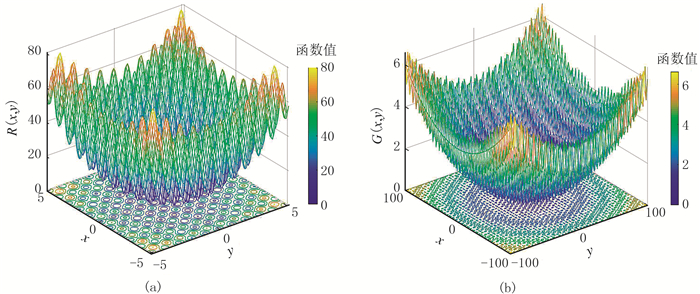图 1 Rastrigin函数(a)和Griewank函数(b)的曲面表 1 三种算法寻优结果对比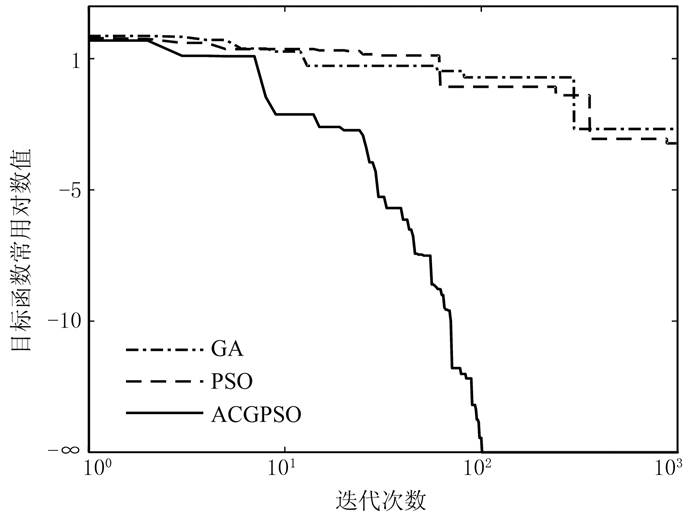图 2 三种算法对Rastrigin函数的寻优迭代对比

2 理论模型试算表 2 模型参数及反演搜索范围
2.1 基阶频散曲线反演测试

 $P(M)=\sqrt{\sum\limits_{i=1}^{N} \frac{\left(V_{i}^{\mathrm{obs}}-V_{i}^{\mathrm{cal}}\right)^{2}}{N}}$ (7)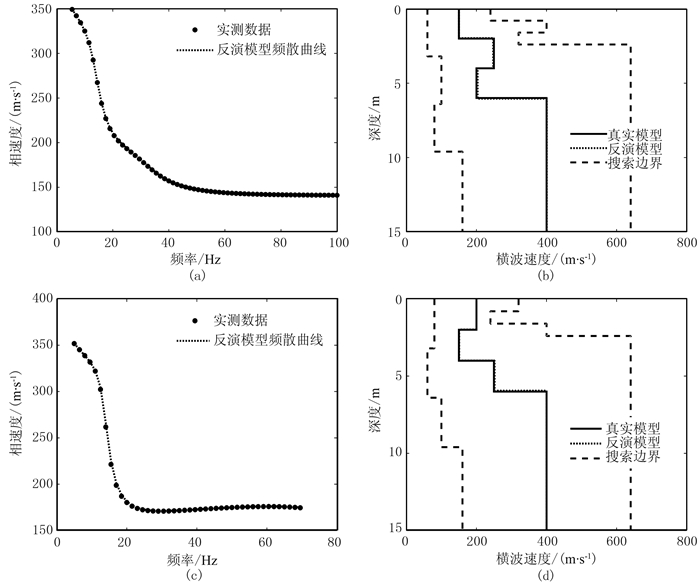图 3 模型A、B不含噪声数据的反演结果 (a)模型A频散曲线；(b)模型A反演的速度剖面；(c)模型B频散曲线；(d)模型B反演的速度剖面表 3 不含噪与含噪数据反演结果统计表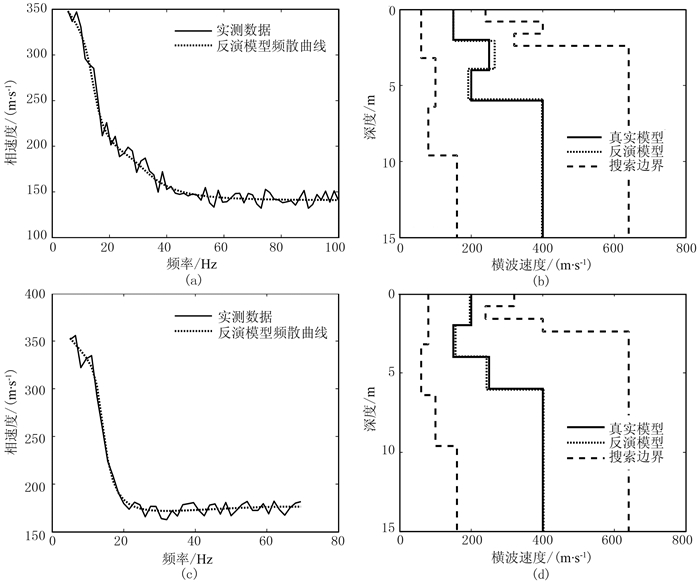图 4 模型A、B含噪声数据的反演结果 (a)模型A频散曲线；(b)模型A反演的速度剖面；(c)模型B频散曲线；(d)模型B反演的速度剖面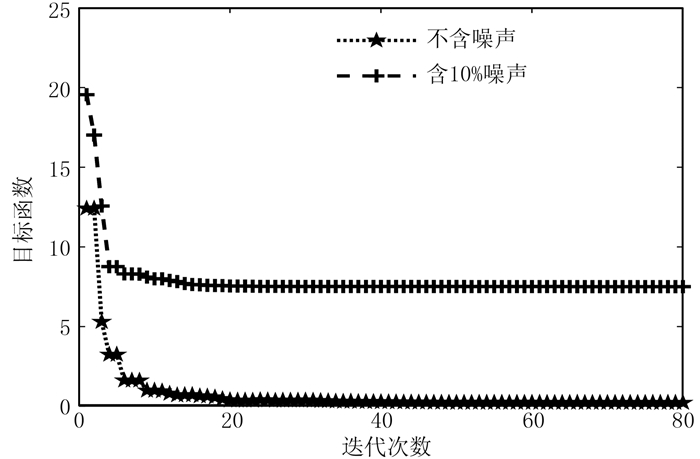图 5 不含噪和含噪时模型A的目标函数变化曲线
2.2 多阶频散曲线联合反演

 $\mathit{\Phi } = \sum\limits_{i = 1}^m {\left\{ {{e_i}\left[ {\frac{1}{{{N_i}}}\sum\limits_{j = 1}^{{N_i}} {{{\left( {V_{i, j}^{{\rm{obs}}} - V_{i, j}^{{\rm{cal}}}} \right)}^2}} } \right]} \right\}}$ (8)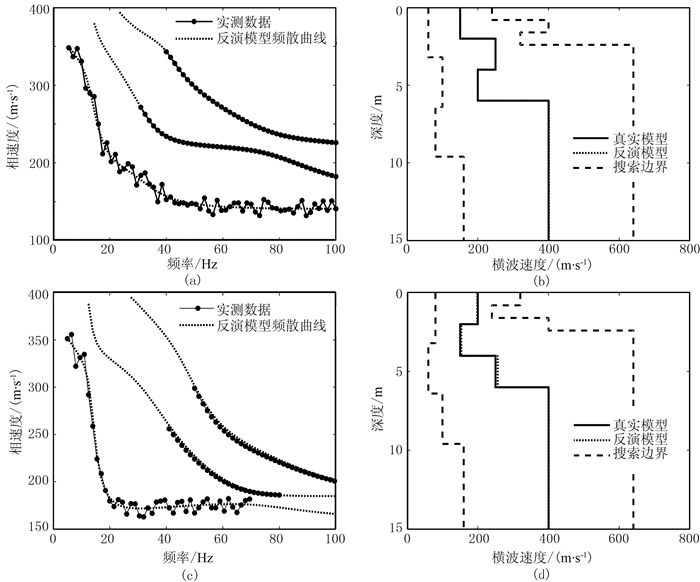图 6 模型A和模型B多阶频散曲线联合反演结果 (a)模型A频散曲线；(b)模型A反演的速度剖面；(c)模型B频散曲线；(d)模型B反演的速度剖面表 4 多阶频散曲线联合反演结果统计表

2.3 与PSO算法反演的对比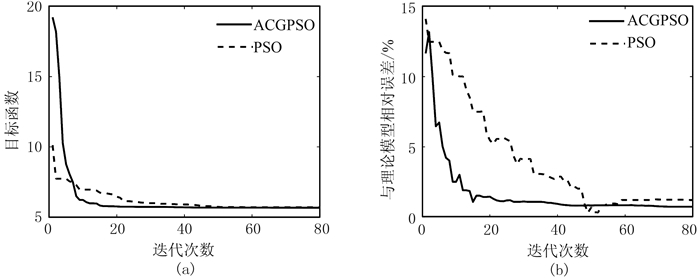图 7 ACGPSO算法与PSO算法对比 (a)目标函数变化曲线；(b)当前最优模型与理论模型的相对误差变化曲线
3 实测数据反演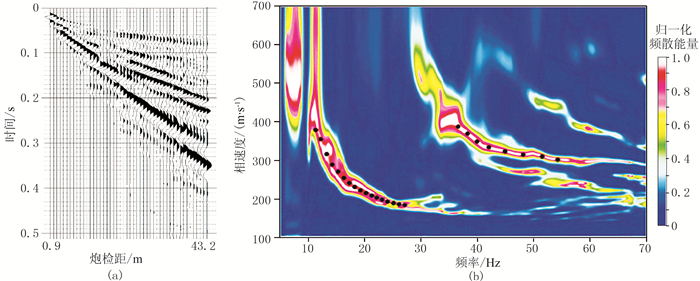图 8 怀俄明地区实际地震数据与频散能量图 (a)实测地震记录；(b)频散能量图表 5 怀俄明M地区反演搜索范围、密度和泊松比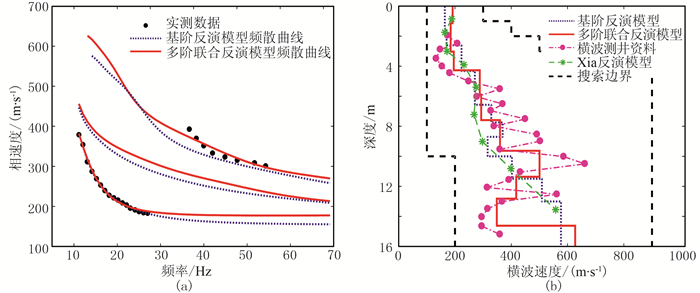图 9 怀俄明M地区频散曲线反演结果 (a)实测数据(圆点)、基阶(虚线)和多阶联合(实线)反演模型频散曲线；(b)横波速度剖面与测井资料
4 结论

(1) ACGPSO算法对两种测试函数均能100%收敛到其理论解，比常规PSO算法和GA算法具有更高的寻优求解能力和收敛速度。

(2) ACGPSO算法对无噪和含噪的频散曲线反演均能取得很好效果，充分表明了该算法的有效性、稳定性和抗噪性，同时也证明基于ACGPSO算法的反演比PSO算法具有更快的收敛速度，更强的稳定性和更高的反演精度。

(3) 两步法反演可较好地判断高阶频散曲线对应的模态，以此进行的多模式联合反演更能反映地下地层真实情况，更具可信度及适用性。

  夏江海. 高频面波方法[M]. 湖北武汉: 中国地质大学出版社, 2015. XIA Jianghai. High-frequency Surface-wave Methods[M]. Wuhan, Hubei: China University of Geosciences Press, 2015.  Park C B, Mille R D, Xia J H. Multichannel analysis of surface wave[J]. Geophysics, 1999, 64(3): 800-808. DOI:10.1190/1.1444590  张碧星, 肖柏勋, 杨文杰, 等. 瑞利波勘探中"之"字型频散曲线的形成机理及反演研究[J]. 地球物理学报, 2000, 43(4): 557-567. ZHANG Bixing, XIAO Baixun, YANG Wenjie, et al. Mechanism of zigzag dispersion curve in Rayleigh exploration and its inversion study[J]. Chinese Journal of Geophysics, 2000, 43(4): 557-567. DOI:10.3321/j.issn:0001-5733.2000.04.017  姜福豪, 李培明, 张翊孟, 等. 多道面波频散分析在实际大炮数据中的应用[J]. 石油地球物理勘探, 2018, 53(1): 17-24, 46. JIANG Fuhao, LI Peiming, ZHANG Yimeng, et al. Frequency dispersion analysis of MASW in real seismic data[J]. Oil Geophysical Prospecting, 2018, 53(1): 17-24, 46.  李子伟, 刘学伟. 空间假频对频率-波数域瑞利面波频散曲线反演的影响[J]. 石油地球物理勘探, 2013, 48(3): 395-402. LI Ziwei, LIU Xuewei. Effects of spatial aliasing on frequency-wavenumber domain inversion of Rayleigh-wave dispersion curve[J]. Oil Geophysical Prospecting, 2013, 48(3): 395-402.  李庆春, 邵广周, 刘金兰, 等. 瑞雷面波勘探的过去、现在和未来[J]. 地球科学与环境学报, 2006, 28(3): 74-77. LI Qingchun, SHAO Guangzhou, LIU Jinlan, et al. Past, present and future of Rayleigh surface wave exploration[J]. Journal of Earth Sciences and Environment, 2006, 28(3): 74-77. DOI:10.3969/j.issn.1672-6561.2006.03.016  Xia J H, Miller R D, Park C B. Estimation of near-surface shear-wave velocity by inversion of Rayleigh waves[J]. Geophysics, 1999, 64(3): 691-700. DOI:10.1190/1.1444578  崔建文. 一种改进的全局优化算法及其在面波频散曲线反演中的应用[J]. 地球物理学报, 2004, 47(3): 521-527. CUI Jianwen. An improved global optimization me-thod and its application to the inversion of surface wave dispersion curve[J]. Chinese Journal of Geophysics, 2004, 47(3): 521-527. DOI:10.3321/j.issn:0001-5733.2004.03.024  曹旭, 熊章强, 张大洲. 基于BP神经网络的瑞雷面波智能优化反演[J]. 工程地球物理学报, 2015, 12(4): 514-519. CAO Xu, XIONG Zhangqiang, ZHANG Dazhou. The Rayleigh surface wave intelligent inversion based on the BP artificial neural network[J]. Chinese Journal of Engineering Geophysics, 2015, 12(4): 514-519. DOI:10.3969/j.issn.1672-7940.2015.04.017  Beaty K S, Schmitt D R, Sacchi M. Simulated annealing inversion of multimode Rayleigh wave dispersion curve for geological structure[J]. Geophysical Journal International, 2002, 151(2): 622-631. DOI:10.1046/j.1365-246X.2002.01809.x  蔡伟, 宋先海, 袁士川, 等. 利用粒子群优化算法快速、稳定反演瑞雷波频散曲线[J]. 石油地球物理勘探, 2018, 53(1): 25-34. CAI Wei, SONG Xianhai, YUAN Shichuan, et al. Fast and stable Rayleigh-wave dispersion-curve inversion based on particle swarm optimization[J]. Oil Geophysical Prospecting, 2018, 53(1): 25-34.  Song X, Gu H, Zhang X, et al. Pattern search algo-rithms for nonlinear inversion of high-frequency Rayleigh wave dispersion curve[J]. Computer & Geosciences, 2008, 34(6): 611-624.  张鑫源, 胡晓敏, 林盈. 遗传算法和粒子群优化算法的性能对比分析[J]. 计算机科学与探索, 2014, 8(1): 90-102. ZHANG Xinyuan, HU Xiaomin, LIN Ying. Comparisons of genetic algorithm and particle swarm optimization[J]. Journal of Frontiers of Computer Science and Technology, 2014, 8(1): 90-102.  Mahor A, Rangnekar S. Short term generation sche-duling of cascadedhydro electric system using novel selfadaptive inertia weight PSO[J]. International Journal of Electrical Power & Energy Systems, 2012, 34(1): 1-9.  Bonyadi M R, Michalewicz Z. A locally convergent rotationally invariant particle[J]. Swarm Intelligence, 2014, 8(3): 159-198. DOI:10.1007/s11721-014-0095-1  Tan Yue, Tan Guanzheng, Deng Shuguang. Hybrid particle swarm optimizationwith chaotic search for solving integer and mixed integer programming problems[J]. Journal of Central South University, 2014, 21(7): 2731-2742. DOI:10.1007/s11771-014-2235-6  Samani M, Tafreshi M, Shafieenejad I, et al. Minimumtime open-loop and closed-loop optimal guidance with GA-PSO and neural fuzzy for Samarai MAV flight[J]. IEEE Aerospace & Electronic Systems Magazine, 2015, 30(5): 28-37.  彭刘亚, 任川. 基于粒子群算法的瑞雷波频散曲线反演研究[J]. 地球物理学进展, 2018, 33(4): 1682-1686. PENG Liuya, REN Chuan. Inversion of Rayleigh wave dispersion curve using particle swarm optimization algorithm[J]. Progress in Geophysics, 2018, 33(4): 1682-1686.  邵广周, 李庆春, 吴华. 基于波场数值模拟的瑞利波频散曲线特征及各模式能量分布[J]. 石油地球物理勘探, 2015, 50(2): 306-315. SHAO Guangzhou, LI Qingchun, WU Hua. Characte-ristics of Rayleigh wave dispersion curve based on wave field numerical simulation and energy distribution of each mode[J]. Oil Geophysical Prospecting, 2015, 50(2): 306-315.  罗银河, 夏江海, 刘江平, 等. 基阶与高阶瑞利波联合反演研究[J]. 地球物理学报, 2008, 51(1): 242-249. LUO Yinhe, XIA Jianghai, LIU Jiangping, et al. Joint inversion of fundamental and higher mode Rayleigh waves[J]. Chinese Journal of Geophysics, 2008, 51(1): 242-249. DOI:10.3321/j.issn:0001-5733.2008.01.030  侯征, 熊盛青, 杨进, 等. 基于人工蜂群算法的瑞雷波多阶模式非线性联合反演研究[J]. 地球物理学进展, 2018, 33(1): 362-371. HOU Zheng, XIONG Shengqing, YANG Jin, et al. Research on nonlinear joint inversion of multimode Rayleigh wave based on artificial bee colony algorithm[J]. Progress in Geophysics, 2018, 33(1): 362-371.  Vinh P C, Malischewsky P G. Improved approxi-mations of the Rayleigh wave velocity[J]. Journal ofThermoplastic Composite Materials, 2008, 21(4): 337-352. DOI:10.1177/0892705708089479  Kennedy J and Eberhart R C.Particle swarm optimization[C].Proceedings of IEEE International Conference on Neural Networks, Perth, 1995, 4, 1942-1948.  龚纯, 王正林. 精通MATLAB优化计算[M]. 第4版. 北京: 电子工业出版社, 2016.  刘雪明, 凡友华, 翟佳羽, 等. 四种典型地层的瑞雷波"之"字型频散曲线数值模拟研究[J]. 地球物理学报, 2009, 52(12): 3042-3050. LIU Xueming, FAN Youhua, ZHAI Jiayu, et al. Numerical simulation of Rayleigh wave zigzag dispersion curve of four typical strata[J]. Chinese Journal of Geophysics, 2009, 52(12): 3042-3050. DOI:10.3969/j.issn.0001-5733.2009.12.013  Xia J, Miller R D, Park C B, et al. A pitfall in shallow shear-wave refraction surveying[J]. Journal of A-pplied Geophysics, 2002, 51(1): 1-9. DOI:10.1016/S0926-9851(02)00197-0